SSC (English Medium) Class 10th Board ExamMaharashtra State Board
Share

# Balbharati solutions for Class 10th Board Exam Algebra chapter 5 - Probability

## Balbharati Class 10 Mathematics 1 Algebra

#### Balbharati Balbharati Class 10 Mathematics 1 Algebra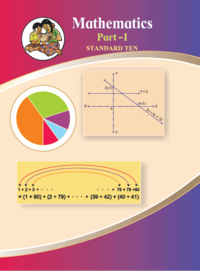## Chapter 5: Probability

#### Chapter 5: Probability solutions [Page 116]

Q 1.1 | Page 116

How many possibilities is the following ?
Vanita knows the following sites in Maharashtra. She is planning to visit one of them in her summer vacation.

Ajintha, Mahabaleshwar, Lonar Sarovar, Tadoba wild life sanctuary, Amboli, Raigad, Matheran, Anandavan.

Q 1.2 | Page 116

How many possibilities is following?

Any day of a week is to be selected randomly.

Q 1.3 | Page 116

How many possibilities is following?

Select one card from the pack of 52 cards.

Q 1.4 | Page 116

How many possibilities is following?

One number from 10 to 20 is written on each card. Select one card randomly.

#### Chapter 5: Probability solutions [Pages 117 - 118]

Q 1.1 | Page 117

For the following experiment write sample space ‘S’ and number of sample points n(S).

One coin and one die are thrown simultaneously.

Q 1.2 | Page 117

For the following experiment write sample space ‘S’ and number of sample points n(S).

Two digit numbers are formed using digits 2, 3 and 5 without repeating a digits.

Q 2 | Page 118

The arrow is rotated and it stops randomly on the disc. Find out on which colour it may stop.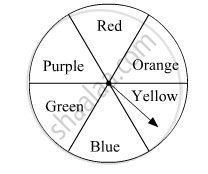Q 3 | Page 118

In the month of March 2019, Find the days on which the date is multiple of 5. (see the given page of the calender)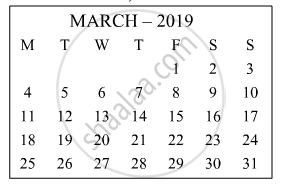Q 4 | Page 118

Form a ‘Road safety commitee’ of two, from 2 boys (B1, B2) and 2 girls(G1, G2). Complete the following activity to write the sample space.
(a) Committee of 2 boys =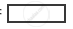b) Committee of 2 girls =(c) Committee of one boy and one girl =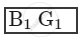∴  Sample space = {..., ..., ..., ..., ..., ...}

#### Chapter 5: Probability solutions [Page 121]

Q 1.1 | Page 121

Write sample space ‘S’ and number of sample point n(S) for the following experiment. Also write events A, B, C in the set form and write n(A), n(B), n(C).
One die is rolled,
Event  A : Even number on the upper face.
Event  B : Odd number on the upper face.
Event  C : Prime number on the upper face.

Q 1.2 | Page 121

Write sample space ‘S’ and number of sample point n(S) for the following experiment. Also write events A, B, C in the set form and write n(A), n(B), n(C).

Two dice are rolled simultaneously,
Event  A : The sum of the digits on upper faces is a multiple of 6.
Event B : The sum of the digits on the upper faces is minimum 10.
Event  C : The same digit on both the upper faces.

Q 1.3 | Page 121

Write sample space ‘S’ and number of sample point n(S) for the following experiment. Also write events A, B, C in the set form and write n(A), n(B), n(C).

Three coins are tossed simultaneously.
Condition for event A : To get at least two heads.
Condition for event B : To get no head.
Condition for event C : To get head on the second coin.

Q 1.4 | Page 121

Write sample space ‘S’ and number of sample point n(S) for the following experiment. Also write events A, B, C in the set form and write n(A), n(B), n(C).

Two digit numbers are formed using digits 0, 1, 2, 3, 4, 5 without repetition of the digits.
Condition for event A : The number formed is even
Condition for event B : The number formed is divisible by 3.
Condition for event C : The number formed is greater than 50.

Q 1.5 | Page 121

Write sample space ‘S’ and number of sample point n(S) for the following experiment. Also write events A, B, C in the set form and write n(A), n(B), n(C).

From three men and two women, environment committee of two persons is to be formed.
Condition for event A : There must be at least one woman member.
Condition for event B : One man, one woman committee to be formed.
Condition for event C : There should not be a woman member.

Q 1.6 | Page 121

Write sample space ‘S’ and number of sample point n(S) for the following experiment. Also write events A, B, C in the set form and write n(A), n(B), n(C).

One coin and one die are thrown simultaneously.
Condition for event A : To get head and an odd number.
Condition for event B : To get a head or tail and an even number.
Condition for event C : Number on the upper face is greater than 7 and tail on the coin.

#### Chapter 5: Probability solutions [Page 125]

Q 1.1 | Page 125

If two coins are tossed, find the probability of the following event.

Q 1.2 | Page 125

If two coins are tossed, find the probability of the following event.

Q 2.1 | Page 125

If two dice are rolled simultaneously, find the probability of the following event.
The sum of the digits on the upper faces is at least 10.

Q 2.2 | Page 125

If two dice are rolled simultaneously, find the probability of the following event.

The sum of the digits on the upper faces is 33.

Q 2.3 | Page 125

If two dice are rolled simultaneously, find the probability of the following event.
The digit on the first die is greater than the digit on second die.

Q 3.1 | Page 125

There are 15 tickets in a box, each bearing one of the numbers from 1 to 15. One ticket is drawn at random from the box. Find the probability of event that the ticket drawn - shows an even number.

Q 3.2 | Page 125

There are 15 tickets in a box, each bearing one of the numbers from 1 to 15. One ticket is drawn at random from the box. Find the probability of event that the ticket drawn - shows a number which is a multiple of 5.

Q 4.1 | Page 125

A two digit number is formed with digits 2, 3, 5, 7, 9 without repetition. What is the probability that the number formed is an odd number ?

Q 4.2 | Page 125

A two digit number is formed with digits 2, 3, 5, 7, 9 without repetition. What is the probability that the number formed is a multiple of 5 ?

Q 5.1 | Page 125

A card is drawn at random from a pack of well shuffled 52 playing cards. Find the probability that the card drawn is – an ace.

Q 5.2 | Page 125

A card is drawn at random from a pack of well shuffled 52 playing cards. Find the probability that the card drawn is – a spade.

#### Chapter 5: Probability solutions [Pages 126 - 128]

Q 1.1 | Page 126

Choose the correct alternative answer for the following question.
Which number cannot represent a probability ?

(A)2/3  (B)1.5  (C) 15%  (D) 0.7

Q 1.2 | Page 126

Choose the correct alternative answer for the following question.

A die is rolled. What is the probability that the number appearing on upper face is less than 3 ?

(A)1/6   (B) 1/3  (C)1/2  (D) 0

Q 1.3 | Page 126

Choose the correct alternative answer for the following question. What is the probability of the event that a number chosen from 1 to 100 is a prime number ?

(A)1/5   (B) 6/25   (C) 1/4   (D) 13/50

Q 1.4 | Page 126

Choose the correct alternative answer for the following question.There are 40 cards in a bag. Each bears a number from 1 to 40. One card is drawn at random. What is the probability that the card bears a number which is a multiple of 5 ?

(A)1/5   (B) 3/5   (C)4/5   (D)1/3

Q 1.5 | Page 126

Choose the correct alternative answer for the following question.If n(A) = 2, P(A) =1/5 , then n(S) = ?

(A)10    (B) 5/2   (C)2/5  (D)1/3

Q 3 | Page 126

In a hockey team there are 6 defenders , 4 offenders and 1 goalee. Out of these, one player is to be selected randomly as a captain. Find the probability of the selection that –
(1) The goalee will be selected.
(2) A defender will be selected.

Q 4 | Page 126

Joseph kept 26 cards in a cap, bearing one English alphabet on each card. One card is drawn at random. What is the probability that the card drawn is a vowel card ?

Q 5 | Page 126

A balloon vendor has 2 red, 3 blue and 4 green balloons. He wants to choose one of them at random to give it to Pranali. What is the probability of the event that Pranali gets,
(1) a red balloon
(2) a blue balloon
(3) a green balloon.

Q 6 | Page 127

A box contains 5 red, 8 blue and 3 green pens. Rutuja wants to pick a pen at random. What is the probability that the pen is blue?

Q 7.1 | Page 127

Six faces of a die are as shown below.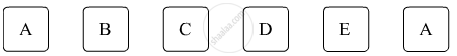If the die is rolled once, find the probability of - ‘A’ appears on upper face.

Q 7.2 | Page 127

Six faces of a die are as shown below.If the die is rolled once, find the probability of -‘D’ appears on upper face.

Q 8.1 | Page 127

A box contains 30 tickets, bearing only one number from 1 to 30 on each. If one ticket is drawn at random, find the probability of an event that the ticket drawn bears an odd number .

Q 8.2 | Page 127

A box contains 30 tickets, bearing only one number from 1 to 30 on each. If one ticket is drawn at random, find the probability of an event that the ticket drawn bears a complete square number.

Q 9 | Page 127

Length and breadth of a rectangular garden are 77 m and 50 m. There is a circular lake in the garden having diameter 14 m. Due to wind, a towel from a terrace on a nearby building fell into the garden. Then find the probability of the event that it fell in the lake.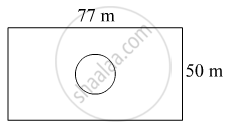Q 10.1 | Page 127

In a game of chance, a spinning arrow comes to rest at one of the numbers 1, 2, 3, 4, 5, 6, 7, 8. All these are equally likely outcomes. Find the probability that it will rest at 8.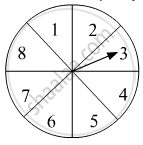Q 10.2 | Page 127

In a game of chance, a spinning arrow comes to rest at one of the numbers 1, 2, 3, 4, 5, 6, 7, 8. All these are equally likely outcomes. Find the probability that it will rest at an odd number.Q 10.3 | Page 127

In a game of chance, a spinning arrow comes to rest at one of the numbers 1, 2, 3, 4, 5, 6, 7, 8. All these are equally likely outcomes. Find the probability that it will rest at a number greater than 2.Q 10.4 | Page 127

In a game of chance, a spinning arrow comes to rest at one of the numbers 1, 2, 3, 4, 5, 6, 7, 8. All these are equally likely outcomes. Find the probability that it will rest at  a number less than 9.Q 11.1 | Page 127

There are six cards in a box, each bearing a number from 0 to 5. Find the probability of the following event, that a card drawn show, a natural number.

Q 11.2 | Page 127

There are six cards in a box, each bearing a number from 0 to 5. Find the probability of the following event, that a card drawn show,  number less than 1.

Q 11.3 | Page 127

There are six cards in a box, each bearing a number from 0 to 5. Find the probability of the following event, that a card drawn show,  a whole number.

Q 11.4 | Page 127

There are six cards in a box, each bearing a number from 0 to 5. Find the probability of the following event, that a card drawn show, a number is greater than 5.

Q 12.1 | Page 128

A bag contains 3 red, 3 white and 3 green balls. One ball is taken out of the bag at random. What is the probability that the ball drawn is – red.

Q 12.2 | Page 128

A bag contains 3 red, 3 white and 3 green balls. One ball is taken out of the bag at random. What is the probability that the ball drawn is –not red.

Q 12.3 | Page 128

A bag contains 3 red, 3 white and 3 green balls. One ball is taken out of the bag at random. What is the probability that the ball drawn is – either red or white.

Q 13 | Page 128

Each card bears one letter from the word ‘mathematics’ The cards are placed on a table upside down. Find the probability that a card drawn bears the letter ‘m’.

Q 14 | Page 128

Out of 200 students from a school, 135 like Kabbaddi and the remaining students do not like the game. If one student is selected at random from all the students, find the probability that the student selected dosen't like Kabbaddi.

Q 15.1 | Page 128

A two digit number is to be formed from the digits 0, 1, 2, 3, 4. Repetition of the digits is allowed. Find the probability that the number so formed is a - prime number.

Q 15.2 | Page 128

A two digit number is to be formed from the digits 0, 1, 2, 3, 4. Repetition of the digits is allowed. Find the probability that the number so formed is a -  multiple of 4.

Q 15.3 | Page 128

A two digit number is to be formed from the digits 0, 1, 2, 3, 4. Repetition of the digits is allowed. Find the probability that the number so formed is a - multiple of 11.

Q 16 | Page 128

The faces of a die bear numbers 0, 1, 2, 3, 4, 5. If the die is rolled twice, then find the probability that the product of digits on the upper face is zero.

Q 17.1 | Page 128

Do the following activity -
Activity I : Total number of students in your class, n(S) =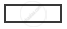Number of students from your class, wearing spectacles, n(A) =Probability of a randomly selected student wearing spectacles, P(A) =Probability of a randomly selected student not wearing spectacles, P(B) =Q 17.2 | Page 128

Do the following activity -

Activity II : Decide the sample space yourself and fill in the following boxes.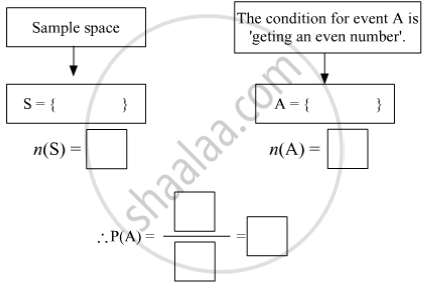## Chapter 5: Probability

#### Balbharati Balbharati Class 10 Mathematics 1 Algebra## Balbharati solutions for Class 10th Board Exam Algebra chapter 5 - Probability

Balbharati solutions for Class 10th Board Exam chapter 5 (Probability) include all questions with solution and detail explanation. This will clear students doubts about any question and improve application skills while preparing for board exams. The detailed, step-by-step solutions will help you understand the concepts better and clear your confusions, if any. Shaalaa.com has the Maharashtra State Board Balbharati Class 10 Mathematics 1 Algebra solutions in a manner that help students grasp basic concepts better and faster.

Further, we at Shaalaa.com provide such solutions so that students can prepare for written exams. Balbharati textbook solutions can be a core help for self-study and acts as a perfect self-help guidance for students.

Concepts covered in Class 10th Board Exam Algebra chapter 5 Probability are Probability Examples and Solutions, Introduction to Probability, Probability - A Theoretical Approach, Type of Event - Elementry, Type of Event - Complementry, Type of Event - Exclusive, Type of Event - Exhaustive, Equally Likely Outcomes, Probability of an Event, Concept Or Properties of Probability, Addition Theorem, Random Experiments, Sample Space.

Using Balbharati Class 10th Board Exam solutions Probability exercise by students are an easy way to prepare for the exams, as they involve solutions arranged chapter-wise also page wise. The questions involved in Balbharati Solutions are important questions that can be asked in the final exam. Maximum students of Maharashtra State Board Class 10th Board Exam prefer Balbharati Textbook Solutions to score more in exam.

Get the free view of chapter 5 Probability Class 10th Board Exam extra questions for and can use Shaalaa.com to keep it handy for your exam preparation

S